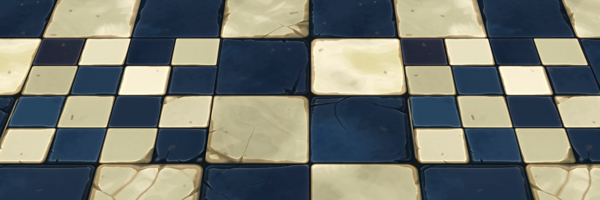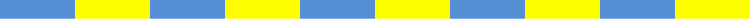# Counting stick challengeHere is a picture of a counting stick:It is divided into equal rectangular sections.

How many rectangles can you see altogether?

Once you've had a go, here are some questions you might like to consider:

• Are all your rectangles the same size? (I can see some that are bigger than others...)
• How many different sizes of rectangle are there?
• How many rectangles are there of each size?
• What if my counting stick was longer, or shorter?
• Is there a quick way to work out how many rectangles there would be, for a counting stick with 100 sections? Or 1000? Or...